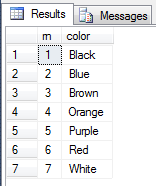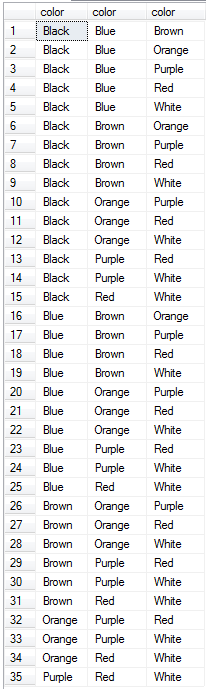﻿ Select Combinations of Data using SQL Query without RepetitionSQL Server and T-SQL Development Tutorials Development resources, articles, tutorials, code samples, tools and downloads for ASP.Net, SQL Server, Reporting Services, T-SQL, Windows, AWS, SAP HANA and ABAP

# Select Combinations of Data using SQL Query

Database developers can build SQL Select query to generate and return all possible combinations of data in SQL Server by using method shown in this SQL tutorial with cross joins and applying a simple logic.
In your application, you might have certain numbers, users, colors that you want to select a number of given items.
For example, from existing 10 items you want to group any 3 of them. So you have exactly (10*9*8)/(3*2) = 120 possible combinations.
Let's create a Transact-SQL query which will return all possible combinations without repetition calculated by the above simple mathematical calculation or formula.

Let's assume in our SQL Server database we have a colors table where a number of color names are stored. Here I share the Create Table DDL statement and the Insert DML commands which will populate the SQL database table with sample data.

Even to make the tutorial sample for combination query, let's insert same colors again in the Colors table.
Our SQL query should take these repeating values as a single data row. Because we will generate combination of these items without repetition.

By using SQL Server DENSE_RANK() window function with Distinct clause, I can easily get a unique list of colors.I will use this colors list from sample database table using SQL CTE expressions and cross join the CTE set 3 times to get a result set for permutation of all available colors in a 3 color slots.

As the count of rows in the returned result set shows (343 rows), SQL programmers will have the permutation of 7 for 3
Or explicitely, by cross joining the table to itself the result set will return all possible permutations of 3 colors (items) from a set of 7
343 rows = 7 * 7 * 7

But our initial requirement for this SQL tutorial is for obtaining the combination of these items not for the permutation.

By applying WHERE clause with suitable criteria, SQL programmers can have the combination set easily in this SQL query.

Now the result set returns "7 choose 3" for combination of 3 colors out of 7 possible without repetition.
Number of rows : (7*6*5)/(3*2*1) = 35Of course the "From clause" and "Where clause" will be modified if you want to choose 4 colors instead of 3.
SQL Server developers will add additional CTE table to the FROM clause using new CROSS JOIN.
And WHERE clause will be modified for the new item.

Here is the SQL combination query for 4 items which has an additional "combination number" field and sorted randomly by the use of SQL NewID() function

For SQL Server developers, it might be also interesting how to calculate factorial of an integer using SQL user-defined function

SQL Server